# Chapter Notes: Rational Numbers Notes | Study Mathematics (Maths) Class 7 - Class 7

## Class 7: Chapter Notes: Rational Numbers Notes | Study Mathematics (Maths) Class 7 - Class 7

The document Chapter Notes: Rational Numbers Notes | Study Mathematics (Maths) Class 7 - Class 7 is a part of the Class 7 Course Mathematics (Maths) Class 7.
All you need of Class 7 at this link: Class 7

9. Rational Numbers

Introduction to Rational Numbers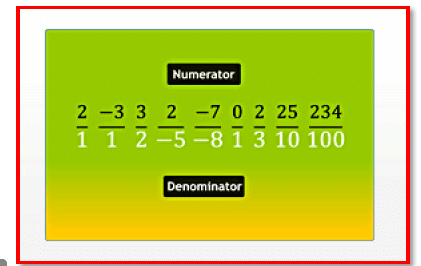All numbers, including whole numbers, integers, fractions and decimal numbers, can be written in the numerator-denominator form.

All numbers, including whole numbers, integers, fractions and decimal numbers, can be written in the numerator-denominator form. A rational number is a number that can be written in the form p/q, where p and q are integers and q ≠ 0.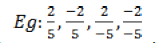The denominator of a rational number can never be zero. A rational number is positive if its numerator and denominator are both either positive integers or negative integers.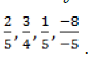If either the numerator or the denominator of a rational number is a negative integer, then the rational number is called a negative rational number.

The rational number zero is neither negative nor positive. On the number line:

o Positive rational numbers are represented to the right of 0.
o Negative rational numbers are represented to the left of 0.

By multiplying or dividing both the numerator and the denominator of a rational number by the same non-zero integer, we can get another rational number that is equivalent to the given rational number.

A rational number is said to be in its standard form if its numerator and denominator have no common factor other than 1, and its denominator is a positive integer.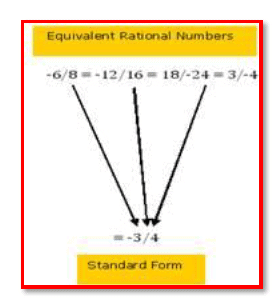Page 35

To reduce a rational number to its standard form, divide its numerator and denominator by their Highest Common Factor (HCF). To find the standard form of a rational number with a negative integer as the denominator, divide its numerator and denominator by their HCF with a minus sign.

Comparison of Rational NumbersWhile comparing positive rational numbers with the same denominator, the number with the greatest numerator is the largest.

While comparing positive rational numbers with the same denominator, the number with the greatest numerator is the largest. It is easy to compare these numbers if their denominators are the same.

Eg: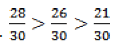A positive rational number is always greater than a negative rational number. While comparing negative rational numbers with the same denominator, compare their numerators ignoring the minus sign. The number with the greatest numerator is the smallest.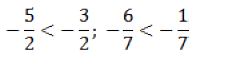Page 36

Positive rational numbers lie to the right of 0, while negative rational numbers lie to the left of 0 on the number line.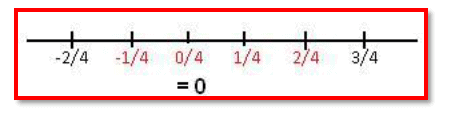To compare rational numbers with different denominators, convert them into equivalent rational numbers with the same denominator, which is equal to the LCM of their denominators. You can find infinite rational numbers between any two given rational numbers.

Operations on Rational Numbers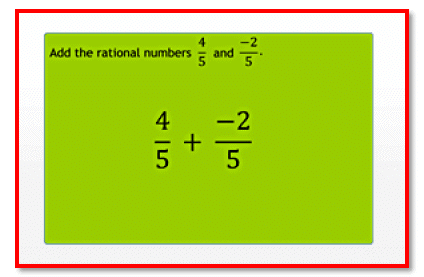To add rational numbers with different denominators

The following points are to be noted while finding the sum of rational numbers:

1. If the denominators of the given rational numbers are the same, then the denominator of their sum will also be the same. The numerator of the sum of two rational numbers with the same denominator is the sum of the numerators of the given numbers.

2. To add rational numbers with different denominators, we convert them into equivalent rational numbers with the same denominator.

3.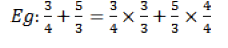Thus, the sum is .

1. Two rational numbers whose sum is zero are called additive inverses of each other.

Page 37

2. The denominator of the difference of two rational numbers with the same denominator is the same as the common denominator of the given numbers.

Subtraction of rational numbers:

1. To subtract rational numbers with different denominators, we must convert them into equivalent rational numbers with the same denominator.

Multiplication of rational numbers:

1. To multiply two rational numbers, we simply multiply their numerators and denominators with their correct signs.

2. Two rational numbers whose product is 1 are called reciprocals of each other.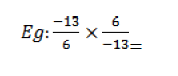3. A rational number and its reciprocal will always have the same sign.

Division of rational numbers:

1. To divide one rational number by another, we actually multiply the first number with the reciprocal of the second number.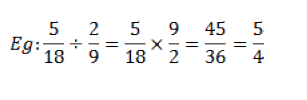The document Chapter Notes: Rational Numbers Notes | Study Mathematics (Maths) Class 7 - Class 7 is a part of the Class 7 Course Mathematics (Maths) Class 7.
All you need of Class 7 at this link: Class 7Use Code STAYHOME200 and get INR 200 additional OFF

## Mathematics (Maths) Class 7

165 videos|252 docs|45 tests

Track your progress, build streaks, highlight & save important lessons and more!

,

,

,

,

,

,

,

,

,

,

,

,

,

,

,

,

,

,

,

,

,

;